# 2.1: Basic Geometric Concepts and Figures

$$\newcommand{\vecs}{\overset { \rightharpoonup} {\mathbf{#1}} }$$ $$\newcommand{\vecd}{\overset{-\!-\!\rightharpoonup}{\vphantom{a}\smash {#1}}}$$$$\newcommand{\id}{\mathrm{id}}$$ $$\newcommand{\Span}{\mathrm{span}}$$ $$\newcommand{\kernel}{\mathrm{null}\,}$$ $$\newcommand{\range}{\mathrm{range}\,}$$ $$\newcommand{\RealPart}{\mathrm{Re}}$$ $$\newcommand{\ImaginaryPart}{\mathrm{Im}}$$ $$\newcommand{\Argument}{\mathrm{Arg}}$$ $$\newcommand{\norm}{\| #1 \|}$$ $$\newcommand{\inner}{\langle #1, #2 \rangle}$$ $$\newcommand{\Span}{\mathrm{span}}$$ $$\newcommand{\id}{\mathrm{id}}$$ $$\newcommand{\Span}{\mathrm{span}}$$ $$\newcommand{\kernel}{\mathrm{null}\,}$$ $$\newcommand{\range}{\mathrm{range}\,}$$ $$\newcommand{\RealPart}{\mathrm{Re}}$$ $$\newcommand{\ImaginaryPart}{\mathrm{Im}}$$ $$\newcommand{\Argument}{\mathrm{Arg}}$$ $$\newcommand{\norm}{\| #1 \|}$$ $$\newcommand{\inner}{\langle #1, #2 \rangle}$$ $$\newcommand{\Span}{\mathrm{span}}$$$$\newcommand{\AA}{\unicode[.8,0]{x212B}}$$

##### Learning Objectives
1. Identify and define points, lines, line segments, rays and planes.
2. Classify angles as acute, right, obtuse, or straight.

### Introduction

You use geometric terms in everyday language, often without thinking about it. For example, any time you say “walk along this line” or “watch out, this road quickly angles to the left” you are using geometric terms to make sense of the environment around you. You use these terms flexibly, and people generally know what you are talking about.

In the world of mathematics, each of these geometric terms has a specific definition. It is important to know these definitions—as well as how different figures are constructed—to become familiar with the language of geometry. Let’s start with a basic geometric figure: the plane.

### Figures on a Plane

A plane is a flat surface that continues forever (or, in mathematical terms, infinitely) in every direction. It has two dimensions: length and width.

You can visualize a plane by placing a piece of paper on a table. Now imagine that the piece of paper stays perfectly flat and extends as far as you can see in two directions, left-to-right and frontto-back. This gigantic piece of paper gives you a sense of what a geometric plane is like: it continues infinitely in two directions. (Unlike the piece of paper example, though, a geometric plane has no height.)

A plane can contain a number of geometric figures. The most basic geometric idea is a point, which has no dimensions. A point is simply a location on the plane. It is represented by a dot. Three points that don’t lie in a straight line will determine a plane.

The image below shows four points, labeled A, B, C, and D.Figure $$\PageIndex{1}$$: A set of points

Two points on a plane determine a line. A line is a one-dimensional figure that is made up of an infinite number of individual points placed side by side. In geometry, all lines are assumed to be straight; if they bend they are called a curve. A line continues infinitely in two directions.

Below is line AB or, in geometric notation, $$\overleftrightarrow{AB}$$. The arrows indicate that the line keeps going forever in the two directions. This line could also be called line BA. While the order of the points does not matter for a line, it is customary to name the two points in alphabetical order.

The image below shows the points A and B and the line $$\overleftrightarrow{AB}$$.Figure $$\PageIndex{2}$$: Line AB
##### Example $$\PageIndex{1}$$

Name the line shown in red.###### Solution

The red line goes through the points C and F, so the line is $$\overleftrightarrow{CF}$$.

Answer: $$\overleftrightarrow{CF}$$

There are two more figures to consider. The section between any two points on a line is called a line segment. A line segment can be very long, very short, or somewhere in between. The difference between a line and a line segment is that the line segment has two endpoints and a line goes on forever. A line segment is denoted by its two endpoints, as in $$\overline{CD}$$.Figure $$\PageIndex{3}$$: Line segment CD

A ray has one endpoint and goes on forever in one direction. Mathematicians name a ray with notation like $$\overrightarrow{EF}$$, where point E is the endpoint and F is a point on the ray. When naming a ray, we always say the endpoint first. Note that $$\overrightarrow{FE}$$ would have the endpoint at F, and continue through E, which is a different ray than $$\overrightarrow{EF}$$, which would have an endpoint at E, and continue through F.

The term “ray” may be familiar because it is a common word in English. “Ray” is often used when talking about light. While a ray of light resembles the geometric term “ray,” it does not go on forever, and it has some width. A geometric ray has no width; only length.

Below is an image of ray EF or $$\overrightarrow{EF}$$. Notice that the endpoint is E.Figure $$\PageIndex{4}$$: Ray EF
##### Example $$\PageIndex{2}$$

Identify each line and line segment in the picture below.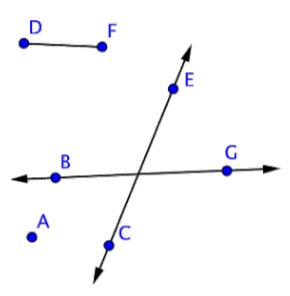###### Solution

Two points define a line, and a line is denoted with arrows. There are two lines in this picture: $$\overleftrightarrow{CE}$$ and $$\overleftrightarrow{BG}$$.

A line segment is a section between two points. $$\overline{DF}$$ is a line segment. But there are also two more line segments on the lines themselves: $$\overline{CE}$$ and $$\overline{BG}$$.

Answer: Lines: $$\overline{CE}$$, $$\overline{BG}$$

Line segments: $$\overline{DF}$$, $$\overline{CE}$$, $$\overline{BG}$$.

##### Example $$\PageIndex{3}$$

Identify each point and ray in the picture below.###### Solution

There are four points: A, B, C, and D. There are also three rays, though only one may be obvious.

Ray $$\overrightarrow{BC}$$ begins at point B and goes through C. Two more rays exist on line $$\overleftrightarrow{AD}$$: they are $$\overrightarrow{DA}$$ and $$\overrightarrow{AD}$$.

Answer: Points: A, B, C, D

Rays: $$\overrightarrow{BC}$$, $$\overrightarrow{AD}$$, $$\overrightarrow{DA}$$

##### Try It Now 1

Which of the following is not represented in the image below?A) BG

B) BA

C) $$\overline{DF}$$

D) AC

### Angles

Lines, line segments, points, and rays are the building blocks of other figures. For example, two rays with a common endpoint make up an angle. The common endpoint of the angle is called the vertex.

The angle ABC is shown below. This angle can also be called ∠ABC, ∠CBA or simply ∠B. When you are naming angles, be careful to include the vertex (here, point B) as the middle letter.The image below shows a few angles on a plane. Notice that the label of each angle is written “point-vertex-point,” and the geometric notation is in the form ∠ABC.Sometimes angles are very narrow; sometimes they are very wide. When people talk about the “size” of an angle, they are referring to the arc between the two rays. The length of the rays has nothing to do with the size of the angle itself. Drawings of angles will often include an arc (as shown above) to help the reader identify the correct ‘side’ of the angle.

Think about an analog clock face. The minute and hour hands are both fixed at a point in the middle of the clock. As time passes, the hands rotate around the fixed point, making larger and smaller angles as they go. The length of the hands does not impact the angle that is made by the hands.

An angle is measured in degrees, represented by the symbol º. A circle is defined as having 360º. (In skateboarding and basketball, “doing a 360” refers to jumping and doing one complete body rotation.

A right angle is any degree that measures exactly 90º. This represents exactly one-quarter of the way around a circle. Rectangles contain exactly four right angles. A corner mark is often used to denote a right angle, as shown in right angle DCB below.

Angles that are between 0º and 90º (smaller than right angles) are called acute angles. Angles that are between 90º and 180º (larger than right angles and less than 180º) are called obtuse angles. And an angle that measures exactly 180º is called a straight angle because it forms a straight line.Figure $$\PageIndex{5}$$: Examples of Angles
##### Example $$\PageIndex{4}$$

Label each angle below as acute, right, or obtuse.###### Solution

You can start by identifying any right angles.

∠GFI is a right angle, as indicated by the corner mark at vertex F.

Acute angles will be smaller than ∠GFI (or less than 90º). This means that ∠DAB and ∠MLN are acute.

∠TQS is larger than ∠GFI, so it is an obtuse angle.

Answer: ∠DAB and ∠MLN are acute angles. ∠GFI is a right angle. ∠TQS is an obtuse angle.

##### Example $$\PageIndex{5}$$

Identify each point, ray, and angle in the picture below.###### Solution

Begin by identifying each point in the figure. There are 4: E, F, G, and J.Now find rays. A ray begins at one point, and then continues through another point towards infinity (indicated by an arrow). Three rays start at point J: $$\overrightarrow{JE}$$, $$\overrightarrow{JF}$$, and $$\overrightarrow{JG}$$. But also notice that a ray could start at point F and go through J and G, and another could start at point G and go through J and F. These rays can be represented by $$\overrightarrow{GF}$$ and $$\overrightarrow{FG}$$.Finally, look for angles. ∠EJG is obtuse, ∠EJF is acute, and ∠FJG is straight. (Don’t forget those straight angles!)Answer: Points: E, F, G, J

Rays: $$\overrightarrow{JE}$$, $$\overrightarrow{JG}$$, $$\overrightarrow{JF}$$, $$\overrightarrow{GF}$$, $$\overrightarrow{FG}$$

Angles: ∠EJG, ∠EJF, ∠FJG

##### Try It Now 2

Identify the acute angles in the given image:### Measuring Angles with a Protractor

Learning how to measure angles can help you become more comfortable identifying the difference between angle measurements. For instance, how is a 135º angle different from a 45º angle?

Measuring angles requires a protractor, which is a semi-circular tool containing 180 individual hash marks. Each hash mark represents 1º. (Think of it like this: a circle is 360º, so a semi-circle is 180º.) To use the protractor, do the following three steps:

Step 1. Line up the vertex of the angle with the dot in the middle of the flat side (bottom) of the protractor,

Step 2. Align one side of the angle with the line on the protractor that is at the zero-degree mark, and

Step 3. Look at the curved section of the protractor to read the measurement.

The example below shows you how to use a protractor to measure the size of an angle.

##### Example $$\PageIndex{6}$$

Use a protractor to measure the angle shown below.###### Solution

Use a protractor to measure the angle.Align the blue dot on the protractor with the vertex of the angle you want to measure.Rotate the protractor around the vertex of the angle until the side of the angle is aligned with the 0 degree mark of the protractor.Read the measurement, in degrees, of the angle. Begin with the side of the angle that is aligned with the 0º mark of the protractor and count up from 0º. This angle measures 38º.##### Try It Now 3

What is the measurement of the angle shown below?### Summary

Geometry begins with simple concepts like points, lines, segments, rays, etc. and expands with angles. As we can see from this section, there are multiple types of angles and several ways to measure them. The most accurate way of measuring an angle is using a protractor. When we put angles together, we obtain geometric shapes and solids, which we discuss in future sections. Next, we discuss lines, and using properties to obtain measures of angles.

1. BA; this image does not show any ray that begins at point B and goes through point A.
2. ∠WAX and ∠YAZ x; both ∠WAX and ∠YAZ are acute angles.
3. 135º; this protractor is aligned correctly, and the correct measurement is 135º.

## 6.1.1: Properties of Angles

##### Learning Objectives
1. Identify parallel and perpendicular lines.
2. Find measures of angles.
3. Identify complementary and supplementary angles.

### Introduction

Imagine two separate and distinct lines on a plane. There are two possibilities for these lines: they will either intersect at one point, or they will never intersect. When two lines intersect, four angles are formed. Understanding how these angles relate to each other can help you figure out how to measure them, even if you only have information about the size of one angle.

### Parallel and Perpendicular

Parallel lines are two or more lines that never intersect. Likewise, parallel line segments are two line segments that never intersect even if the line segments were turned into lines that continued forever. Examples of parallel line segments are all around you, in the two sides of this page and in the shelves of a bookcase. When you see lines or structures that seem to run in the same direction, never cross one another, and are always the same distance apart, there’s a good chance that they are parallel.

Perpendicular lines are two lines that intersect at a 90º (right) angle. And perpendicular line segments also intersect at a 90º (right) angle. You can see examples of perpendicular lines everywhere as well—on graph paper, in the crossing pattern of roads at an intersection, to the colored lines of a plaid shirt. In our daily lives, you may be happy to call two lines perpendicular if they merely seem to be at right angles to one another. When studying geometry, however, you need to make sure that two lines intersect at a 90º angle before declaring them to be perpendicular.

The image below shows some parallel and perpendicular lines. The geometric symbol for parallel is ||, so you can show that AB || CD. Parallel lines are also often indicated by the marking >> on each line (or just a single > on each line). Perpendicular lines are indicated by the symbol ⊥, so you can write $$\overleftrightarrow{WX} ⊥ \overleftrightarrow{YZ}$$.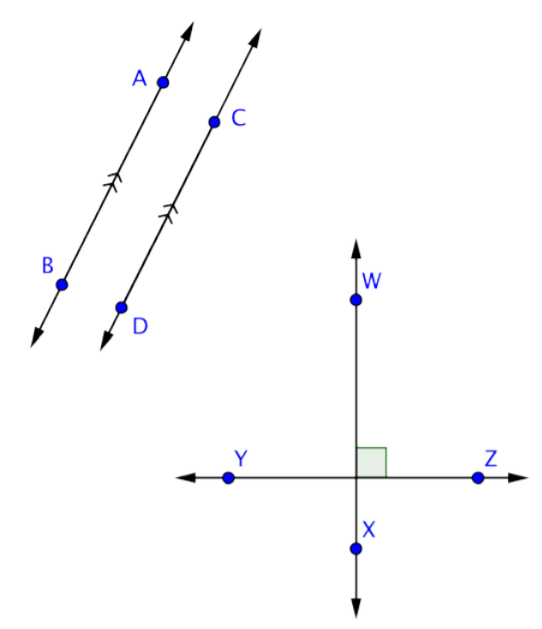If two lines are parallel, then any line that is perpendicular to one line will also be perpendicular to the other line. Similarly, if two lines are both perpendicular to the same line, then those two lines are parallel to each other. Let’s take a look at one example and identify some of these types of lines.

##### Example $$\PageIndex{7}$$

Identify a set of parallel lines and a set of perpendicular lines in the image below.###### Solution

Parallel lines never meet, and perpendicular lines intersect at a right angle. $$\overleftrightarrow{AB}$$ and $$\overleftrightarrow{CD}$$ do not intersect in this image, but if you imagine extending both lines, they will intersect soon. So, they are neither parallel nor perpendicular.$$\overleftrightarrow{AB}$$ is perpendicular to both $$\overleftrightarrow{WX}$$ and $$\overleftrightarrow{YZ}$$, as indicated by the right-angle marks at the intersection of those lines.Since $$\overleftrightarrow{AB}$$ is perpendicular to both lines, then $$\overleftrightarrow{WX}$$ and $$\overleftrightarrow{YZ}$$ are parallel.Answer: $$\overleftrightarrow{WX}$$ || $$\overleftrightarrow{YZ}$$

$$\overleftrightarrow{AB}$$ ⊥ $$\overleftrightarrow{WX}$$, $$\overleftrightarrow{AB}$$ ⊥ $$\overleftrightarrow{YZ}$$

##### Try It Now 1

Which statement most accurately represents the image below?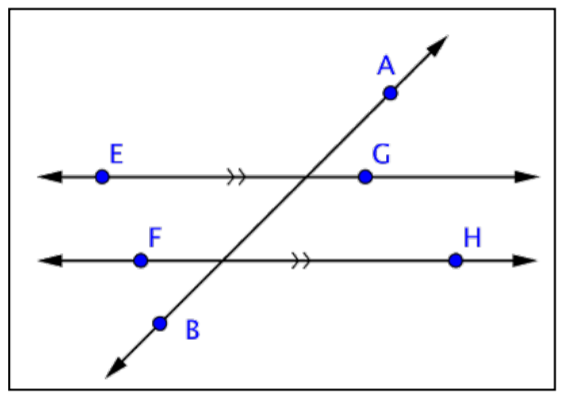A) EF || GH

B) AB ⊥ EG

C) FH || EG

D) AB || FH

### Finding Angle Measurements

Understanding how parallel and perpendicular lines relate can help you figure out the measurements of some unknown angles. To start, all you need to remember is that perpendicular lines intersect at a 90º angle and that a straight angle measures 180º.

The measure of an angle such as ∠A is written as m∠A. Look at the example below. How can you find the measurements of the unmarked angles?

##### Example $$\PageIndex{8}$$

Find the measurement of ∠IJF.###### Solution

Only one angle, ∠HJM, is marked in the image. Notice that it is a right angle, so it measures 90º. ∠HJM is formed by the intersection of lines $$\overleftrightarrow{IM}$$ and $$\overleftrightarrow{HF}$$. Since $$\overleftrightarrow{IM}$$ is a line, ∠IJM is a straight angle measuring 180º.You can use this information to find the measurement of ∠HJI :

m∠HJM + m∠HJI = m∠IJM

90º + m∠HJI = 180º

m∠HJI = 90ºNow use the same logic to find the measurement of ∠IJF. ∠IJF is formed by the intersection of lines $$\overleftrightarrow{IM}$$ and $$\overleftrightarrow{HF}$$. Since $$\overleftrightarrow{HF}$$ is a line, ∠HJF will be a straight angle measuring 180º.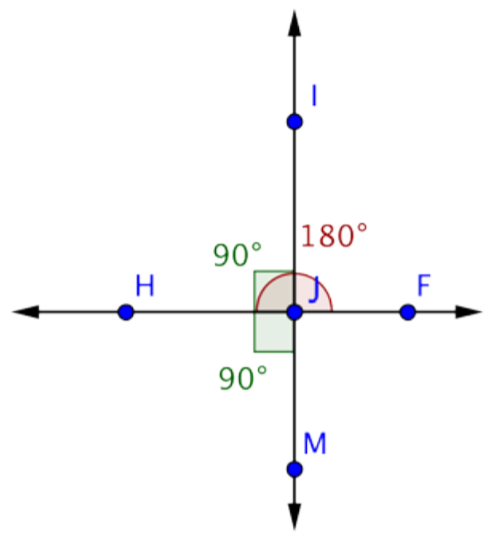You know that ∠HJI measures 90º. Use this information to find the measurement of ∠IJF:

m∠HJM + m∠IJF = m∠HJF

90º + m∠IJF = 180º

m∠IJF = 90ºIn this example, you may have noticed that angles ∠HJI, ∠IJF, and ∠HJM are all right angles. (If you were asked to find the measurement of ∠FJM, you would find that angle to be 90º, too.) This is what happens when two lines are perpendicular—the four angles created by the intersection are all right angles.

Not all intersections happen at right angles, though. In the example below, notice how you can use the same technique as shown above (using straight angles) to find the measurement of a missing angle.

##### Example $$\PageIndex{9}$$

Find the measurement of ∠DAC.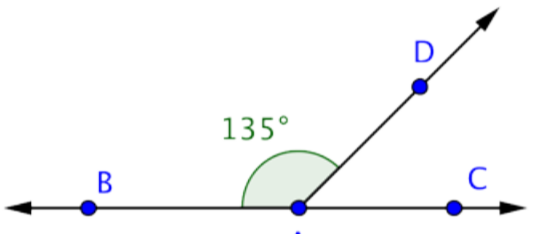###### Solution

This image shows the line $$\overleftrightarrow{BC}$$ and the ray $$\overrightarrow{AD}$$ intersecting at point A. The measurement of ∠BAD is 135º. You can use straight angles to find the measurement of ∠DAC.∠BAC is a straight angle, so it measures 180º.Use this information to find the measurement of ∠DAC.

135º + m∠DAC = 180º

m∠DAC = 45º##### Try It Now 2### Supplementary and Complementary

In the example above, m∠BAC and m∠DAC add up to 180º. Two angles whose measures add up to 180º are called supplementary angles. There’s also a term for two angles whose measurements add up to 90º, they are called complementary angles.

One way to remember the difference between the two terms is that “corner” and “complementary” each begin with c (a 90º angle looks like a corner), while straight and “supplementary” each begin with s (a straight angle measures 180º).

If you can identify supplementary or complementary angles within a problem, finding missing angle measurements is often simply a matter of adding or subtracting.

##### Example $$\PageIndex{10}$$

Two angles are supplementary. If one of the angles measures 48º, what is the measurement of the other angle?

###### Solution

Two supplementary angles make up a straight angle, so the measurements of the two angles will be 180º.

m∠A + m∠B = 180º

You know the measurement of one angle. To find the measurement of the second angle, subtract 48º from 180º.

48º+ m∠B = 180º

m∠B = 180º - 48º

m∠B = 132º

Answer: The measurement of the other angle is 132º

##### Example $$\PageIndex{11}$$

Find the measurement of ∠AXZ.###### Solution

This image shows two intersecting lines, $$\overleftrightarrow{AB}$$ and $$\overleftrightarrow{YZ}$$. They intersect at point X, forming four angles. Angles ∠AXY and ∠AXZ are supplementary because together they make up the straight angle ∠YXZ.Use this information to find the measurement of ∠AXZ.

m∠AXY + m∠AXZ = m∠YXZ

30º + m∠AXZ = 180º

m∠AXZ = 150º##### Example $$\PageIndex{12}$$

Find the measurement of ∠BAC.###### Solution

This image shows the line $$\overleftrightarrow{CF}$$ and the rays $$\overleftrightarrow{AB}$$ and $$\overleftrightarrow{AD}$$, all intersecting at point A. Angle ∠BAD is a right angle. Angles ∠BAC and ∠CAD are complementary because together they create ∠BAD.Use this information to find the measurement of ∠BAC .

m∠BAC + 50º = 90º

m∠BAC = 40º##### Example $$\PageIndex{13}$$###### Solution

You know the measurements of two angles here: ∠CAB and ∠DAE. You also know that m∠BAE = 180º.Use this information to find the measurement of ∠CAD.

m∠BAC + m∠CAD + m∠DAE = m∠BAE

25º + m∠CAD + 75º = 180º##### Try It Now 3

Which pair of angles is complementary?A) ∠PKO and ∠MKN

B) ∠PKO and ∠PKM

C) ∠LKP and ∠LKN

D) ∠LKM and ∠MKN

### Summary

Parallel lines do not intersect, while perpendicular lines cross at a 90º angle. Two angles whose measurements add up to 180º are said to be supplementary, and two angles whose measurements add up to 90º are said to be complementary. For most pairs of intersecting lines, all you need is the measurement of one angle to find the measurements of all other angles formed by the intersection.

1. C) FH || EG; both EG and FH are marked with >> on each line, and those markings mean they are parallel.
2. 137º; ∠BAD is a straight angle measuring 180º. Since ∠BAC measures 43º, the measure of ∠CAD must be 180º – 43º = 137º.
3. D) ∠LKM and ∠MKN; the measurements of two complementary angles will add up to 90º. ∠LKP is a right angle, so ∠LKN must be a right angle as well. ∠LKM + ∠MKN = ∠LKN, so ∠LKM and ∠MKN are complementary.

## 6.1.2: Triangles

##### Learning Objectives
1. Identify equilateral, isosceles, scalene, acute, right, and obtuse triangles.
2. Identify whether triangles are similar, congruent, or neither.
3. Identify corresponding sides of congruent and similar triangles.
4. Find the missing measurements in a pair of similar triangles.
5. Solve application problems involving similar triangles

### Introduction

Geometric shapes, also called figures, are an important part of the study of geometry. The triangle is one of the basic shapes in geometry. It is the simplest shape within a classification of shapes called polygons. All triangles have three sides and three angles, but they come in many different shapes and sizes. Within the group of all triangles, the characteristics of a triangle’s sides and angles are used to classify it even further. Triangles have some important characteristics, and understanding these characteristics allows you to apply the ideas in real-world problems.

### Classifying and Naming Triangles

A polygon is a closed plane figure with three or more straight sides. Polygons each have a special name based on the number of sides they have. For example, the polygon with three sides is called a triangle because “tri” is a prefix that means “three.” Its name also indicates that this polygon has three angles. The prefix “poly” means many.

The table below shows and describes three classifications of triangles. Notice how the types of angles in the triangle are used to classify the triangle.

 Name of Triangle Picture of Triangle Description Acute TriangleA triangle with 3 acute angles (3 angles measuring between 0° and 90°). Obtuse TriangleA triangle with 1 obtuse angle (1 angle measuring between 90° and 180°). Right TriangleA triangle containing one right angle (1 angle that measures 90°). Note that the right angle is shown with a corner mark and does not need to be labeled 90°.

The sum of the measures of the three interior angles of a triangle is always 180°. This fact can be applied to find the measure of the third angle of a triangle, if you are given the other two. Consider the examples below.

##### Example $$\PageIndex{14}$$

A triangle has two angles that measure 35° and 75°. Find the measure of the third angle.

###### Solution

The sum of the three interior angles of a triangle is 180°.

35° + 75° + $$x$$ = 180°

Find the value of $$x$$.

110º + $$x$$ = 180º

$$x$$ = 180° ‒ 110º

$$x$$ = 70°

Answer: The third angle of the triangle measures 70°.

##### Example $$\PageIndex{15}$$

One of the angles in a right triangle measures 57º. Find the measurement of the third angle.

###### Solution

The sum of the three angles of a triangle is 180°. One of the angles has a measure of 90° as it is a right triangle.

57° + 90° + $$x$$ = 180°

Simplify.

147º + $$x$$ = 180°

Find the value of $$x$$.

$$x$$ = 180º - 147º

$$x$$ = 33º

Answer: The third angle of the right triangle measures 33°.

There is an established convention for naming triangles. The labels of the vertices of the triangle, which are generally capital letters, are used to name a triangle.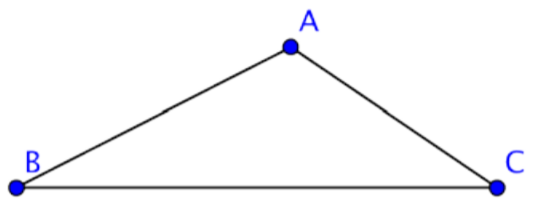You can call this triangle ABC or ∆ABC since A, B, and C are vertices of the triangle. When naming the triangle, you can begin with any vertex. Then keep the letters in order as you go around the polygon. The triangle above could be named in a variety of ways: ∆ABC, or ∆CBA. The sides of the triangle are line segments AB, AC, and CB.

Just as triangles can be classified as acute, obtuse, or right based on their angles, they can also be classified by the length of their sides. Sides of equal length are called congruent sides. While we designate a segment joining points A and B by the notation $$\overline{AB}$$, we designate the length of a segment joining points A and B by the notation AB without a segment bar over it. The length AB is a number, and the segment $$\overline{AB}$$ is the collection of points that make up the segment.

Mathematicians show congruency by putting a hash mark symbol through the middle of sides of equal length. If the hash mark is the same on one or more sides, then those sides are congruent. If the sides have different hash marks, they are not congruent. The table below shows the classification of triangles by their side lengths.

 Name of Triangle Picture of Triangle Description Equilateral TriangleA triangle whose three sides have the same length. These sides of equal length are called congruent sides. Isosceles TriangleA triangle with exactly two congruent sides. Scalene TriangleA triangle in which all three sides are a different length.

To describe a triangle even more specifically, you can use information about both its sides and its angles. Consider this example.

##### Example $$\PageIndex{16}$$

Classify the triangle below.###### Solution

Notice what kind of angles the triangle has. Since one angle is a right angle, this is a right triangle.Notice the lengths of the sides. Are there congruence marks or other labels?The congruence marks tell us there are two sides of equal length. So, this is an isosceles triangle.

Answer: This is an isosceles right triangle

##### Try It Now 1

Classify the given triangle.### Identifying Congruent and Similar Triangles

Two triangles are congruent if they are exactly the same size and shape. In congruent triangles, the measures of corresponding angles and the lengths of corresponding sides are equal. Consider the two triangles shown below:Since both ∠B and ∠E are right angles, these triangles are right triangles. Let’s call these two triangles ∆ABC and ∆DEF. These triangles are congruent if every pair of corresponding sides has equal lengths and every pair of corresponding angles has the same measure.

The corresponding sides are opposite the corresponding angles.

↔ means “corresponds to”

∠B ↔ ∠E

∠A ↔ ∠D

∠C ↔ ∠F

$$\overline{AB}$$ ↔ $$\overline{DE}$$

$$\overline{AC}$$ ↔ $$\overline{DF}$$

$$\overline{BC}$$ ↔ $$\overline{EF}$$

∆ABC and ∆DEF are congruent triangles as the corresponding sides and corresponding angles are equal.

Let’s take a look at another pair of triangles. Below are the triangles ∆ABC and ∆RST.These two triangles are surely not congruent because ∆RST is clearly smaller in size than ∆ABC. But, even though they are not the same size, they do resemble one another. They are the same shape. The corresponding angles of these triangles look like they might have the same exact measurement, and if they did they would be congruent angles and we would call the triangles similar triangles.

Congruent angles are marked with hash marks, just as congruent sides are.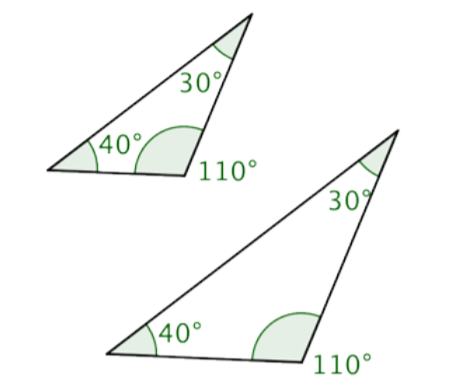Figure $$\PageIndex{6}$$: Image showing angle measurements of both triangles.Figure $$\PageIndex{7}$$: Image showing triangles ABC and RST using hash marks to show angle congruency.

We can also show congruent angles by using multiple bands within the angle, rather than multiple hash marks on one band. Below is an image using multiple bands within the angle.Figure $$\PageIndex{8}$$: Image showing triangles ABC and RST using bands to show angle congruency.

If the corresponding angles of two triangles have the same measurements they are called similar triangles. This name makes sense because they have the same shape, but not necessarily the same size. When a pair of triangles is similar, the corresponding sides are proportional to one another. That means that there is a consistent scale factor that can be used to compare the corresponding sides. In the previous example, the side lengths of the larger triangle are all 1.4 times the length of the smaller. So, similar triangles are proportional to one another.

Just because two triangles look similar does not mean they are similar triangles in the mathematical sense of the word. Checking that the corresponding angles have equal measure is one way of being sure the triangles are similar.

### Corresponding Sides of Similar Triangles

There is another method for determining similarity of triangles that involves comparing the ratios of the lengths of the corresponding sides.

If the ratios of the pairs of corresponding sides are equal, the triangles are similar.

Consider the two triangles below.∆ABC is not congruent to ∆DEF because the side lengths of ∆DEF are longer than those of ∆ABC. So, are these triangles similar? If they are, the corresponding sides should be proportional.

Since these triangles are oriented in the same way, you can pair the left, right, and bottom sides: $$\overline{AB}$$ and $$\overline{DE}$$, $$\overline{BC}$$ and $$\overline{EF}$$, $$\overline{AC}$$ and $$\overline{DF}$$. (You might call these the two shortest sides, the two longest sides, and the two leftover sides and arrive at the same ratios). Now we will look at the ratios of their lengths.

$$\dfrac{\overline{AB}}{\overline{DE}} = \dfrac{\overline{BC}}{\overline{EF}} = \dfrac{\overline{AC}}{\overline{DF}}$$

Substituting the side length values into the proportion, you see that it is true:

$$\dfrac{3}{9} = \dfrac{4}{12} = \dfrac{6}{18}$$

If the corresponding sides are proportional, then the triangles are similar. Triangles ABC and DEF are similar, but not congruent.

Let’s use this idea of proportional corresponding sides to determine whether two more triangles are similar.

##### Example $$\PageIndex{17}$$

Determine if the triangles below are similar by seeing if their corresponding sides are proportional.###### Solution

First determine the corresponding sides, which are opposite corresponding angles.

$$\overline{CA}$$ ↔ $$\overline{FD}$$

$$\overline{AB}$$ ↔ $$\overline{DE}$$

$$\overline{BC}$$ ↔ $$\overline{EF}$$

Write the corresponding side lengths as ratios.

$$\dfrac{\overline{CA}}{\overline{FD}} = \dfrac{\overline{AB}}{\overline{DE}} = \dfrac{\overline{BC}}{\overline{EF}}$$

Substitute the side lengths into the ratios, and determine if the ratios of the corresponding sides are equivalent. They are, so the triangles are similar.

$$\dfrac{10}{5} = \dfrac{6}{3} = \dfrac{14}{7}$$

$$2 = 2 = 2$$

Answer: ∆ABC and ∆DEF are similar.

The mathematical symbol ~ means “is similar to”. So, you can write ∆ABC is similar to ∆DEF as ∆ABC ~ ∆DEF.

##### Try It Now 2

Determine whether the two triangles are similar, congruent, or neither.### Finding Missing Measurements in Similar Triangles

You can find the missing measurements in a triangle if you know some measurements of a similar triangle. Let’s look at an example.

##### Example $$\PageIndex{18}$$

∆ABC and ∆XYZ are similar triangles. What is the length of side BC?###### Solution

In similar triangles, the ratios of corresponding sides are proportional. Set up a proportion of two ratios, one that includes the missing side.

$$\dfrac{BC}{YZ} = \dfrac{AB}{XY}$$

Substitute in the known side lengths for the side names in the ratio. Let the unknown side length be n.

$$\dfrac{n}{2} = \dfrac{6}{1.5}$$

Solve for n using cross multiplication.

$$2 \cdot 6 = 1.5 \cdot n$$

$$12 = 1.5n$$

$$8 = n$$

This process is fairly straightforward—but be careful that your ratios represent corresponding sides, recalling that corresponding sides are opposite corresponding angles.

### Solving Application Problems Involving Similar Triangles

Applying knowledge of triangles, similarity, and congruence can be very useful for solving problems in real life. Just as you can solve for missing lengths of a triangle drawn on a page, you can use triangles to find unknown distances between locations or objects.

Let’s consider the example of two trees and their shadows. Suppose the sun is shining down on two trees, one that is 6 feet tall and the other whose height is unknown. By measuring the length of each shadow on the ground, you can use triangle similarity to find the unknown height of the second tree.

First, let’s figure out where the triangles are in this situation. The trees themselves create one pair of corresponding sides. The shadows cast on the ground are another pair of corresponding sides. The third side of these imaginary similar triangles runs from the top of each tree to the tip of its shadow on the ground. This is the hypotenuse of the triangle.

If you know that the trees and their shadows form similar triangles, you can set up a proportion to find the height of the tree.

##### Example $$\PageIndex{19}$$

When the sun is at a certain angle in the sky, a 6-foot tree will cast a 4-foot shadow. How tall is a tree that casts an 8-foot shadow?###### Solution

The angle measurements are the same, so the triangles are similar triangles. Since they are similar triangles, you can use proportions to find the size of the missing side.

$$\dfrac{\text{Tree 1}}{\text{Tree 2}} = \dfrac{\text{Shadow 1}}{\text{Shadow 2}}$$

Set up a proportion comparing the heights of the trees and the lengths of their shadows.

Substitute in the known lengths. Call the missing tree height h.

$$\dfrac{6}{h} = \dfrac{4}{8}$$

Solve for h using cross-multiplication.

$$6 \cdot 8 = 4h$$

$$48 = 4h$$

$$12 = h$$

Answer: The tree is 12 feet tall.

### Summary

Triangles are one of the basic shapes in the real world. Triangles can be classified by the characteristics of their angles and sides, and triangles can be compared based on these characteristics. The sum of the measures of the interior angles of any triangle is 180º. Congruent triangles are triangles of the same size and shape. They have corresponding sides of equal length and corresponding angles of the same measurement. Similar triangles have the same shape, but not necessarily the same size. The lengths of their sides are proportional. Knowledge of triangles can be a helpful in solving real-world problems.

1. Obtuse scalene; this triangle has vertices P, Q, and R, one angle (angle Q) that is between 90º and 180º, and sides of three different lengths.

2. ∆ABC and ∆DEF are neither similar nor congruent; the corresponding angle measures are not known to be equal as shown by the absence of congruence marks on the angles. Also, the ratios of the corresponding sides are not equal: $$\dfrac{6.5}{5} = \dfrac{6.5}{5} \neq \dfrac{5}{5}$$

## 6.1.3: Pythagorean Theorem

##### Learning Objectives
1. Use the Pythagorean Theorem to find the unknown side of a right triangle.
2. Solve application problems involving the Pythagorean Theorem.

### Introduction

A long time ago, a Greek mathematician named Pythagoras discovered an interesting property about right triangles: the sum of the squares of the lengths of each of the triangle’s legs is the same as the square of the length of the triangle’s hypotenuse. This property—which has many applications in science, art, engineering, and architecture—is now called the Pythagorean Theorem.

Let’s take a look at how this theorem can help you learn more about the construction of triangles. And the best part—you don’t even have to speak Greek to apply Pythagoras’ discovery.

### The Pythagorean Theorem

Pythagoras studied right triangles, and the relationships between the legs and the hypotenuse of a right triangle, before deriving his theory.##### The Pythagorean Theorem

If a and b are the lengths of the legs of a right triangle and c is the length of the hypotenuse, then the sum of the squares of the lengths of the legs is equal to the square of the length of the hypotenuse.

This relationship is represented by the formula: $$a^2 + b^2 = c^2$$

In the box above, you may have noticed the word “square,” as well as the small 2s to the top right of the letters in $$a^2 + b^2 = c^2$$. To square a number means to multiply it by itself. So, for example, to square the number $$5$$ you multiply $$5 \cdot 5$$, and to square the number $$12$$, you multiply $$12 \cdot 12$$. Some common squares are shown in the table below.

 Number Number Times Itself Square 1 $$1^2 = 1 \cdot 1$$ 1 2 $$2^2 = 2 \cdot 2$$ 4 3 $$3^2 = 3 \cdot 3$$ 9 4 $$4^2 = 4 \cdot 4$$ 16 5 $$5^2 = 5 \cdot 5$$ 25 10 $$10^2 = 10 \cdot 10$$ 100

When you see the equation $$a^2 + b^2 = c^2$$, you can think of this as “the length of side a times itself, plus the length of side b times itself is the same as the length of side c times itself.”

Let’s try out all of the Pythagorean Theorem with an actual right triangle.This theorem holds true for this right triangle—the sum of the squares of the lengths of both legs is the same as the square of the length of the hypotenuse. And, in fact, it holds true for all right triangles.

The Pythagorean Theorem can also be represented in terms of area. In any right triangle, the area of the square drawn from the hypotenuse is equal to the sum of the areas of the squares that are drawn from the two legs. You can see this illustrated below in the same 3-4-5 right triangle.Note that the Pythagorean Theorem only works with right triangles.

### Finding the Length of the Hypotenuse

You can use the Pythagorean Theorem to find the length of the hypotenuse of a right triangle if you know the length of the triangle’s other two sides, called the legs. Put another way, if you know the lengths of a and b, you can find c.In the triangle above, you are given measures for legs a and b: 5 and 12, respectively. You can use the Pythagorean Theorem to find a value for the length of c, the hypotenuse.

The Pythagorean Theorem.

$$a^2 + b^2 = c^2$$

Substitute known values for a and b.

$$(5)^2 + (12)^2 = c^2$$

Evaluate.

$$25 + 144 = c^2$$

Simplify. To find the value of c, think about a number that, when multiplied by itself, equals 169. Does 10 work? How about 11? 12? 13? (You can use a calculator to multiply if the numbers are unfamiliar.)

$$169 = c^2$$

The square root of 169 is 13.

$$c = 13$$

Using the formula, you find that the length of c, the hypotenuse, is 13.

In this case, you did not know the value of c—you were given the square of the length of the hypotenuse, and had to figure it out from there. When you are given an equation like $$169 = c^2$$ and are asked to find the value of c, this is called finding the square root of a number. (Notice you found a number, c, whose square was 169.)

Finding a square root takes some practice, but it also takes knowledge of multiplication, division, and a little bit of trial and error. Look at the table below.

 Number $$x$$ Number $$y$$ which, when multiplied by itself, equals number $$x$$ Square Root $$y$$ 1 $$1 \cdot 1$$ 1 4 $$2 \cdot 2$$ 2 9 $$3 \cdot 3$$ 3 16 $$4 \cdot 4$$ 4 25 $$5 \cdot 5$$ 5 100 $$10 \cdot 10$$ 10

It is a good habit to become familiar with the squares of the numbers from 0‒10, as these arise frequently in mathematics. If you can remember those square numbers—or if you can use a calculator to find them—then finding many common square roots will be just a matter of recall.

##### Try It Now 1

For which of these triangles is $$(3)^2 + (3)^2 = r^2$$?

A)B)C)D)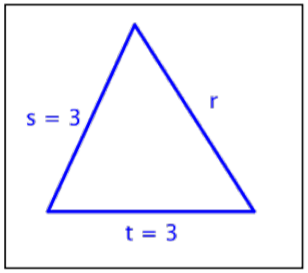### Finding the Length of a Leg

You can use the same formula to find the length of a right triangle’s leg if you are given measurements for the lengths of the hypotenuse and the other leg. Consider the example below.

##### Example $$\PageIndex{20}$$

Find the length of side a in the triangle below. Use a calculator to estimate the square root to one decimal place.###### Solution

In this right triangle, you are given the measurements for the hypotenuse, c, and one leg, b. The hypotenuse is always opposite the right angle and it is always the longest side of the triangle.

$$a$$ = ?

$$b$$ = 6

$$c$$ = 7

To find the length of leg a, substitute the known values into the Pythagorean Theorem.

$$a^2 + b^2 = c^2$$

$$a^2 + 6^2 = 7^2$$

Solve for $$a^2$$. Think: what number, when added to 36, gives you 49?

$$a^2 + 36 = 49$$

$$a^2 = 13$$

Use a calculator to find the square root of 13. The calculator gives an answer of 3.6055…, which you can round to 3.6. (Since you are approximating, you use the symbol ≈.)

$$a ≈ 3.6$$

##### Try It Now 2

Which of the following correctly uses the Pythagorean Theorem to find the missing side, $$x$$?A) $$8^2 + 10^2 = x^2$$

B) $$x + 8 = 10$$

C) $$x^2 + 8^2 = 10^2$$

D) $$x^2 + 10^2 = 8^2$$

### Using the Pythagorean Theorem to Solve Real-world Problems

The Pythagorean Theorem is perhaps one of the most useful formulas you will learn in mathematics because there are so many applications of it in real world settings. Architects and engineers use this formula extensively when building ramps, bridges, and buildings. Look at the following examples.

##### Example $$\PageIndex{21}$$

The owners of a house want to convert a stairway leading from the ground to their back porch into a ramp. The porch is 3 feet off the ground, and due to building regulations, the ramp must start 12 feet away from the base of the porch. How long will the ramp be?

Use a calculator to find the square root, and round the answer to the nearest tenth.

###### Solution

To solve a problem like this one, it often makes sense to draw a simple diagram showing where the legs and hypotenuse of the triangle lie.Identify the legs and the hypotenuse of the triangle. You know that the triangle is a right triangle since the ground and the raised portion of the porch are perpendicular—this means you can use the Pythagorean Theorem to solve this problem. Identify a, b, and c.

$$a$$ = 3

$$b$$ = 12

$$c$$ = ?

Use the Pythagorean Theorem to find the length of c.

$$a^2 + b^2 = c^2$$

$$3^2 + 12^2 = c^2$$

$$9 + 144 = c^2$$

$$153 = c^2$$

Use a calculator to find c.

$$12.4 = c^2$$

The square root of 153 is 12.369…, so you can round that to 12.4.

Answer: The ramp will be 12.4 feet long.

##### Example $$\PageIndex{22}$$

A sailboat has a large sail in the shape of a right triangle. The longest edge of the sail measures 17 yards, and the bottom edge of the sail is 8 yards. How tall is the sail?

###### Solution

Draw an image to help you visualize the problem. In a right triangle, the hypotenuse will always be the longest side, so here it must be 17 yards. The problem also tells you that the bottom edge of the triangle is 8 yards.Setup the Pythagorean Theorem.

$$a^2 + b^2 = c^2$$

$$a^2 + 8^2 = 17^2$$

$$a^2 + 64 = 289$$

$$a^2 = 225$$

$$15 \cdot 15 = 225$$, so

$$a = 15$$

Answer: The height of the sail is 15 yards.

### Summary

The Pythagorean Theorem states that in any right triangle, the sum of the squares of the lengths of the triangle’s legs is the same as the square of the length of the triangle’s hypotenuse. This theorem is represented by the formula 222 abc + = . Put simply, if you know the lengths of two sides of a right triangle, you can apply the Pythagorean Theorem to find the length of the third side. Remember, this theorem only works for right triangles.

1. B); this is a right triangle; when you sum the squares of the lengths of the sides, you get the square of the length of the hypotenuse.
2. C) $$x^2 + 8^2 = 10^2$$; in this triangle, the hypotenuse has length $$10$$, and the legs have length $$8$$ and $$x$$. Substituting into the Pythagorean Theorem you have: $$x^2 + 8^2 = 10^2$$; this equation is the same as $$x^2 + 64 = 100$$, or $$x^2 = 36$$. What number, times itself, equals $$36$$? That would make $$x = 6$$.